• 支持向量机的相关经典案例，里面包含线性核函数和非线性核函数，另外还有实例：支持向量机手写数字识别；内含测试集训练集、代码源文件及注释，可直接运行（需安装numpy和matplotlib）
• %matplotlib inline import matplotlib.pyplot as plt import numpy as np def plot_hyperplane(clf, X, y, h=0.02, draw_sv=True, title='hype...
%matplotlib inline
import matplotlib.pyplot as plt
import numpy as np
def plot_hyperplane(clf, X, y,
h=0.02,
draw_sv=True,
title='hyperplan'):
# create a mesh to plot in
x_min, x_max = X[:, 0].min() - 1, X[:, 0].max() + 1
y_min, y_max = X[:, 1].min() - 1, X[:, 1].max() + 1
xx, yy = np.meshgrid(np.arange(x_min, x_max, h),
np.arange(y_min, y_max, h))

plt.title(title)
plt.xlim(xx.min(), xx.max())
plt.ylim(yy.min(), yy.max())
plt.xticks(())
plt.yticks(())

Z = clf.predict(np.c_[xx.ravel(), yy.ravel()])
# Put the result into a color plot
Z = Z.reshape(xx.shape)
plt.contourf(xx, yy, Z, cmap='hot', alpha=0.5)

markers = ['o', 's', '^']
colors = ['b', 'r', 'c']
labels = np.unique(y)
for label in labels:
plt.scatter(X[y==label][:, 0],
X[y==label][:, 1],
c=colors[label],
marker=markers[label])
if draw_sv:
sv = clf.support_vectors_
plt.scatter(sv[:, 0], sv[:, 1], c='y', marker='x')
from sklearn import svm
from sklearn.datasets import make_blobs

X, y = make_blobs(n_samples=100, centers=2,
random_state=0, cluster_std=0.3)
clf = svm.SVC(C=1.0, kernel='linear')
clf.fit(X, y)

plt.figure(figsize=(12, 4), dpi=144)
plot_hyperplane(clf, X, y, h=0.01,
title='Maximum Margin Hyperplan')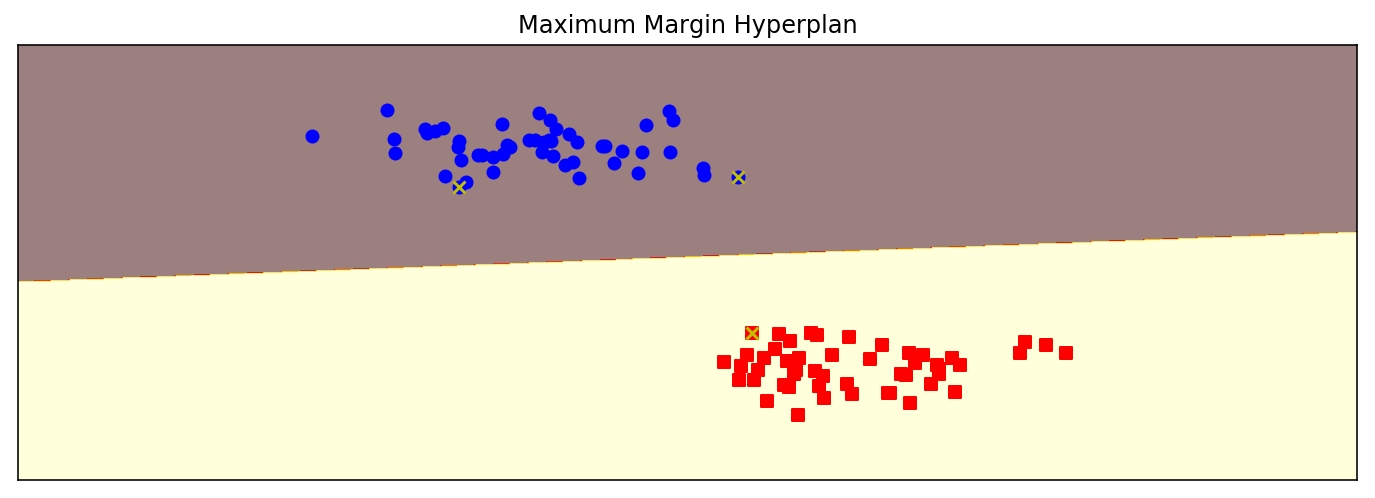from sklearn import svm
from sklearn.datasets import make_blobs

X, y = make_blobs(n_samples=100, centers=3,
random_state=0, cluster_std=0.8)
clf_linear = svm.SVC(C=1.0, kernel='linear')
clf_poly = svm.SVC(C=1.0, kernel='poly', degree=3)
clf_rbf = svm.SVC(C=1.0, kernel='rbf', gamma=0.5)
clf_rbf2 = svm.SVC(C=1.0, kernel='rbf', gamma=0.1)

plt.figure(figsize=(10, 10), dpi=144)

clfs = [clf_linear, clf_poly, clf_rbf, clf_rbf2]
titles = ['Linear Kernel',
'Polynomial Kernel with Degree=3',
'Gaussian Kernel with $\gamma=0.5$',
'Gaussian Kernel with $\gamma=0.1$']
for clf, i in zip(clfs, range(len(clfs))):
clf.fit(X, y)
plt.subplot(2, 2, i+1)
plot_hyperplane(clf, X, y, title=titles[i])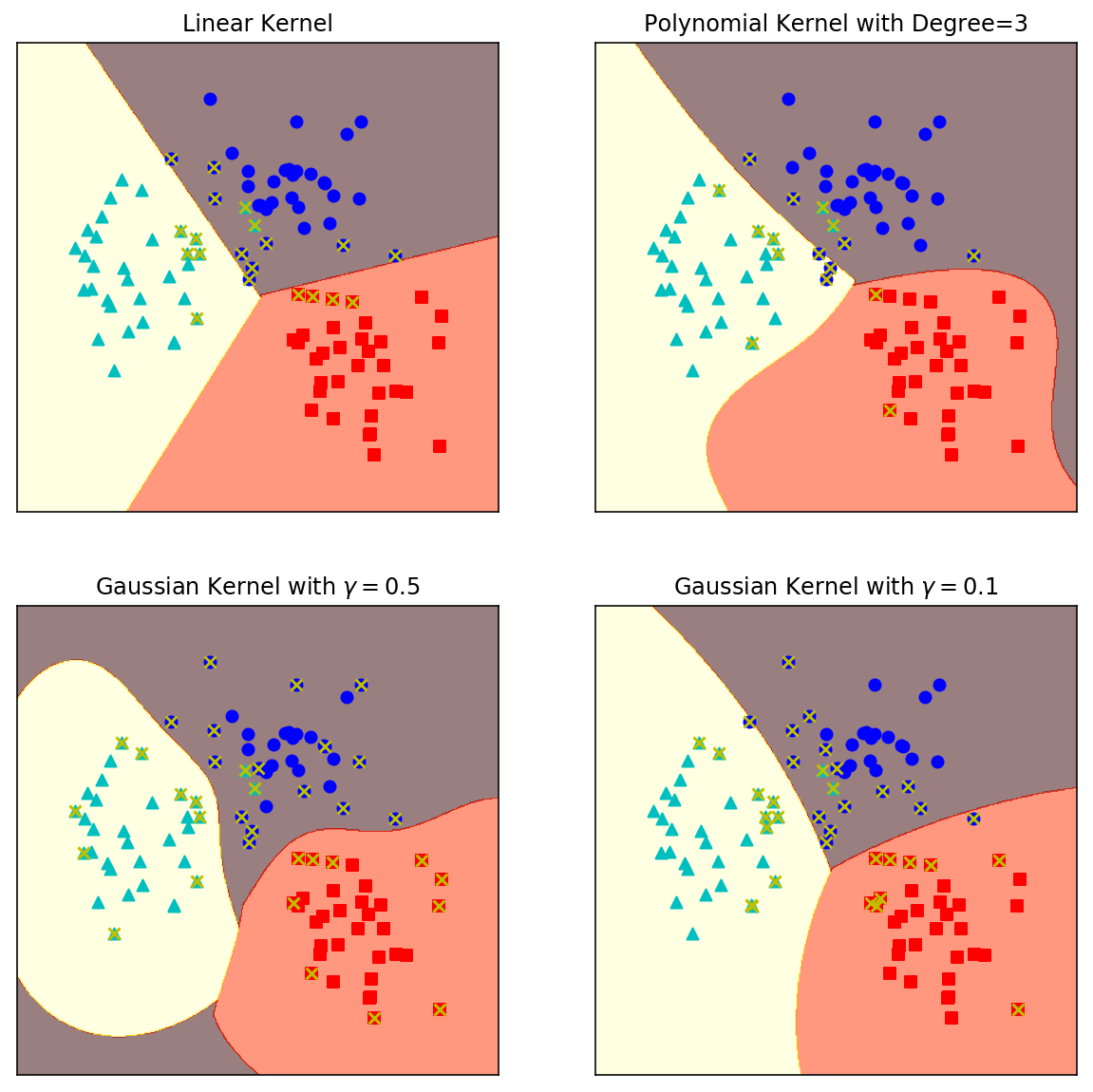展开全文• 总结一下遇到的各种核函数~

千次阅读 2018-08-07 12:50:12
由于之前做了很多核方法相关...首先，再对核方法的思想进行描述，核函数的思想是一个伟大的想法，它工作简练巧妙的映射，解决了高维空间中数据量庞大的问题，在机器学习中是对算法进行非线性改进的利器。如下，...

https://blog.csdn.net/bbbeoy/article/details/72461886

由于之前做了很多核方法相关的子空间学习算法，本文打算对各种核函数进行一下简要的介绍，希望对大家能够有所帮助。

首先，再对核方法的思想进行描述，核函数的思想是一个伟大的想法，它工作简练巧妙的映射，解决了高维空间中数据量庞大的问题，在机器学习中是对算法进行非线性改进的利器。如下，如果在原空间中，给定的样本数据X是线性不可分的，那么如果我们能够将数据映射到高维空间中，即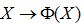那么在高维空间中，样本数据很有可能线性可分，这个特点可以用下图做一个很好的说明：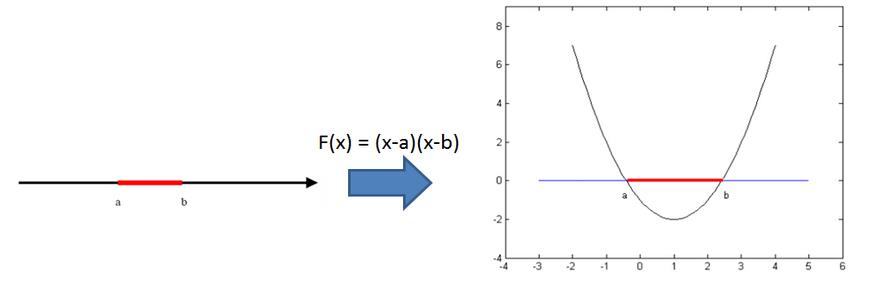如左图，红色部分的点为一类数据，黑色部分的点为另一类，在一维空间中，你不可能通过一刀切将两类数据分开，至少需要两刀。OK，这就说明数据分布是非线性的，我们采用高维映射，当然了，例子中只是映射到了二维空间，但已经足够说明问题了，在右图中，完全可以通过沿着X轴方向的一刀切将两类数据分开，说明在二维空间中，数据已经变成线性可分的了。

这个时候，我们就可以采用很多已有的线性算法对数据进行处理，但是问题来了，映射函数具体形式是什么？这个问题的答案是，根本不需要知道映射函数的具体形式，直接对高维数据进行操作吧！

比如说最经典的PCA算法，这部分内容其实是对前一篇帖子的回顾（http://blog.csdn.net/wsj998689aa/article/details/40398777

PCA在原始空间中的数学模型如下所示：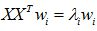在高维空间中的形式就是：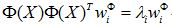我们对其进行简单的变换，我们知道，一个空间中的基向量完全可以用所有的训练样本线性表示出来，即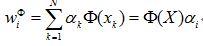见证神奇的时刻到了，上式带入上上式，就可以得到如下形式：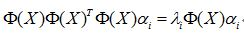如果你再在等号两侧同时左乘一个东西，就会变成如下形式：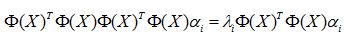核方法的价值在于如下等式成立，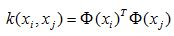请注意，这个公式表达的意思只是高维空间中向量的内积等于原空间中向量之间的核函数值，根核函数的具体形式没有一毛钱的关系。我们继续，一个样本是这样的，那么矩阵化的形式如下：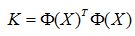那么PCA就变成了如下形式：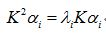看到没，核方法借用核函数的性质，将高维映射成功的抹掉了。那么什么样的函数才可以被称作为核函数呢？这里大牛们给出了一个著名的定理，称作mercer定理。

Mercer 定理：任何半正定的函数都可以作为核函数。所谓半正定的函数f(xi,xj)，是指拥有训练数据集合（x1,x2,...xn)，我们定义一个矩阵的元素aij = f(xi,xj)，这个矩阵式n*n的，如果这个矩阵是半正定的，那么f(xi,xj)就称为半正定的函数。

请注意，这个mercer定理不是核函数必要条件，只是一个充分条件，即还有不满足mercer定理的函数也可以是核函数。所谓半正定指的就是核矩阵K的特征值均为非负。

本文将遇到的核函数进行收集整理，分享给大家。

1. Linear Kernel

线性核是最简单的核函数，核函数的数学公式如下：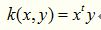如果我们将线性核函数应用在KPCA中，我们会发现，推导之后和原始PCA算法一模一样，很多童鞋借此说“kernel is shit！！！”，这是不对的，这只是线性核函数偶尔会出现等价的形式罢了。

2. Polynomial Kernel

多项式核实一种非标准核函数，它非常适合于正交归一化后的数据，其具体形式如下：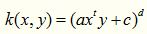这个核函数是比较好用的，就是参数比较多，但是还算稳定。

3. Gaussian Kernel

这里说一种经典的鲁棒径向基核，即高斯核函数，鲁棒径向基核对于数据中的噪音有着较好的抗干扰能力，其参数决定了函数作用范围，超过了这个范围，数据的作用就“基本消失”。高斯核函数是这一族核函数的优秀代表，也是必须尝试的核函数，其数学形式如下：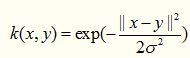虽然被广泛使用，但是这个核函数的性能对参数十分敏感，以至于有一大把的文献专门对这种核函数展开研究，同样，高斯核函数也有了很多的变种，如指数核，拉普拉斯核等。

4. Exponential Kernel

指数核函数就是高斯核函数的变种，它仅仅是将向量之间的L2距离调整为L1距离，这样改动会对参数的依赖性降低，但是适用范围相对狭窄。其数学形式如下：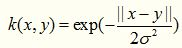5. Laplacian Kernel

拉普拉斯核完全等价于指数核，唯一的区别在于前者对参数的敏感性降低，也是一种径向基核函数。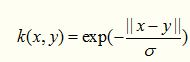6. ANOVA Kernel

ANOVA 核也属于径向基核函数一族，其适用于多维回归问题，数学形式如下：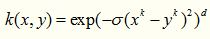7. Sigmoid Kernel

Sigmoid 核来源于神经网络，现在已经大量应用于深度学习，是当今机器学习的宠儿，它是S型的，所以被用作于“激活函数”。关于这个函数的性质可以说好几篇文献，大家可以随便找一篇深度学习的文章看看。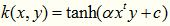二次有理核完完全全是作为高斯核的替代品出现，如果你觉得高斯核函数很耗时，那么不妨尝试一下这个核函数，顺便说一下，这个核函数作用域虽广，但是对参数十分敏感，慎用！！！！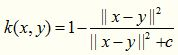多元二次核可以替代二次有理核，它是一种非正定核函数。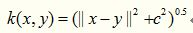顾名思义，逆多元二次核来源于多元二次核，这个核函数我没有用过，但是据说这个基于这个核函数的算法，不会遇到核相关矩阵奇异的情况。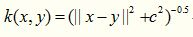11. Circular Kernel

这个核函数没有用过，其数学形式如下所示：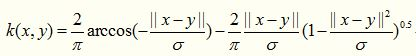12. Spherical Kernel

这个核函数是上一个的简化版，形式如下所示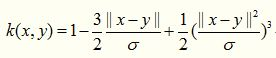13. Wave Kernel

这个核函数没有用过，其适用于语音处理场景。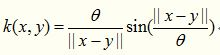14. Triangular  Kernel

三角核函数感觉就是多元二次核的特例，数学公式如下：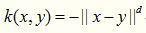15. Log Kernel

对数核一般在图像分割上经常被使用，数学形式如下：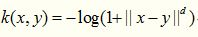16. Spline Kernel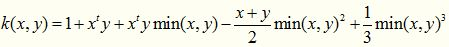17. Bessel Kernel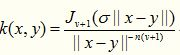18. Cauchy Kernel

柯西核来源于神奇的柯西分布，与柯西分布相似，函数曲线上有一个长长的尾巴，说明这个核函数的定义域很广泛，言外之意，其可应用于原始维度很高的数据上。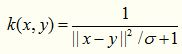19. Chi-Square Kernel

卡方核，这是我最近在使用的核函数，让我欲哭无泪，在多个数据集上都没有用，竟然比原始算法还要差劲，不知道为什么文献作者首推这个核函数，其来源于卡方分布，数学形式如下：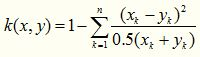它存在着如下变种：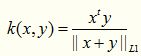其实就是上式减去一项得到的产物，这个核函数基于的特征不能够带有赋值，否则性能会急剧下降，如果特征有负数，那么就用下面这个形式：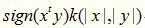20. Histogram Intersection Kernel

直方图交叉核在图像分类里面经常用到，比如说人脸识别，适用于图像的直方图特征，例如extended LBP特征其数学形式如下，形式非常的简单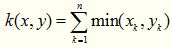21. Generalized Histogram Intersection

顾名思义，广义直方图交叉核就是上述核函数的拓展，形式如下：22. Generalized T-Student Kernel

TS核属于mercer核，其数学形式如下，这个核也是经常被使用的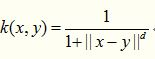23. Bayesian Kernel

贝叶斯核函数还没有用到过。

展开全文机器学习 核函数
• 关于SVM中线性核函数和高斯核函数的选择1.基本数据准备2.各情况对比1. SVM(kernel='linear')：直接采用数据集[966,1850]2. SVM(kernel='rbf')：直接采用数据集[966,1850]3. LR：直接采用数据集[966,1850]4. 降维之后...

关于SVM中线性核函数和高斯核函数的选择

SVM中常用核函数一般是线性核函数和高斯核函数。以sklearn中的SVC，提供的’linear’和’rbf’做说明。面向[n,m]原始数据集，一般的选取准则：

1. 相对于n，m很大。比如m≥n, m=10000, n=10~1000,即(m/n)>10。
考虑’linear’
2. m很小，n一般大小。比如m=1-1000, n=10~10000,即(m/n)在[0.0001,100].
考虑’rbf’
3. m很小，n很大。比如n=1-1000，m=50000+，即(m/n)在[~,0.02].
增加m的量，考虑’linear’

补充：logistic约等同于’linear’的选择

#!/usr/bin/python
# encoding: utf-8

"""
@author : jack_lu
@File : SVM
@time : 2018/12/12 12:12
"""

# 练习所用数据集
from sklearn.datasets import fetch_lfw_people,olivetti_faces

# 特征提取方法
from sklearn.decomposition import PCA
from sklearn.discriminant_analysis import LinearDiscriminantAnalysis
# 特征转换方法
from sklearn.preprocessing import StandardScaler

# sklearn模型方法
from sklearn.model_selection import train_test_split

# metric方法
from sklearn.metrics import accuracy_score

# 机器学习模型
from sklearn.linear_model import LogisticRegression
from sklearn.pipeline import Pipeline
from sklearn.svm import SVC

1.基本数据准备

print('#'*50 + '  1.基本数据准备  ' + '#'*50)
lfw_people2 = fetch_lfw_people(min_faces_per_person=70, resize=0.4)  # 需要通过翻墙下载，C:\Users\Administrator\scikit_learn_data\lfw_home\joblib\sklearn\datasets\lfw\_fetch_lfw_people

##################################################  1.基本数据准备  ##################################################

n_samples, h, w = lfw_people2.images.shape
X = lfw_people2.data
y = lfw_people2.target
n_features = X.shape

target_names = lfw_people2.target_names
n_class = target_names.shape

print('#'*20,'数据集基本情况','#'*20)
print('**样本数量**：%d' %(X.shape))
print('**特征维度**：%d' %(X.shape))
print('**目标类别数**：%d' %(n_class))
print('#'*20,'数据集基本情况','#'*20)

#################### 数据集基本情况 ####################
**样本数量**：1288
**特征维度**：1850
**目标类别数**：7
#################### 数据集基本情况 ####################

X_train,X_test,y_train,y_test = train_test_split(X, y, test_size=0.25,random_state=1)

print('#'*20,'训练数据集基本情况','#'*20)
print('**训练样本数量**：%d' %(X_train.shape))
print('**训练特征维度**：%d' %(X_train.shape))
print('**目标类别数**：%d' %(n_class))
print('#'*20,'训练数据集基本情况','#'*20)

#################### 训练数据集基本情况 ####################
**训练样本数量**：966
**训练特征维度**：1850
**目标类别数**：7
#################### 训练数据集基本情况 ####################

2.各情况对比

print('#'*50 + '  2.建模与比较  ' + '#'*50)

##################################################  2.建模与比较  ##################################################

1. SVM(kernel=‘linear’)：直接采用数据集[966,1850]

svm_origin = SVC(kernel='linear', C=1000, decision_function_shape='ovo')  # 根据官方说明，对于多分类任务宜采用'ovo'即onevsone策略
svm_origin.fit(X_train, y_train)
y_pred = svm_origin.predict(X_test)
print('**情况1-linear的准确率**: %s' %(accuracy_score(y_pred=y_pred, y_true=y_test)))

**情况1-linear的准确率**: 0.832298136646

2. SVM(kernel=‘rbf’)：直接采用数据集[966,1850]

svm_rbf = SVC(kernel='rbf', C=1000, decision_function_shape='ovo')  # 根据官方说明，对于多分类任务宜采用'ovo'即onevsone策略
svm_rbf.fit(X_train, y_train)
y_pred = svm_rbf.predict(X_test)
print('**情况2-rbf的准确率**: %s' %(accuracy_score(y_pred=y_pred, y_true=y_test)))

**情况2-rbf的准确率**: 0.44099378882

3. LR：直接采用数据集[966,1850]

lr_origin = LogisticRegression()  # 对于多分类任务，multi_class可选择'ovr'或者'auto'自动选择，这里按照默认'auto'
lr_origin.fit(X_train, y_train)
y_pred = lr_origin.predict(X_test)
print('**情况3-LR的准确率**: %s' %(accuracy_score(y_pred=y_pred, y_true=y_test)))

**情况3-LR的准确率**: 0.826086956522

4. 降维之后

print('#'*20,'维度由1850减少到150之后','#'*20)

#################### 维度由1850减少到150之后 ####################

def namestr(obj, namespace):
return [name for name in namespace if namespace[name] is obj]
print(namestr(lr_origin,globals()),'\n',
namestr(lr_origin,globals()))

['lr_origin', 'model']
lr_origin

def small_feature_model(model,X_train=X_train,y_train=y_train,X_test=X_test, y_test=y_test):
pca = PCA(n_components=150,random_state=0,whiten=True)
pipeline = Pipeline([('scale',StandardScaler()),('pca',pca)])
processing = pipeline.fit(X_train)
X_train = processing.transform(X_train)
X_test = processing.transform(X_test)
model.fit(X_train, y_train)
y_pred = model.predict(X_test)
#     print(namestr(model,globals()))
print('**small-%s的准确率**: %.3f' %(namestr(model, globals()),accuracy_score(y_pred=y_pred, y_true=y_test)))

for model in [svm_origin, svm_rbf, lr_origin]:
small_feature_model(model)

**small-svm_origin的准确率**: 0.789
**small-svm_rbf的准确率**: 0.811
**small-lr_origin的准确率**: 0.835

print('#'*50 + '  完成  ' + '#'*50)

##################################################  完成  ##################################################

3.小结

从结果看到：

1. 将维度减少到150之后，选择kernel='rbf’的效果>‘linear’;
2. 在没有调参情况下，LR的效果还不错,初期建模值得先尝试。

当然，上面是指定了特定的参数，更主要的目的是对比SVM两种核方法在n和m的比例当中的效果。在具体问题当中，在计算力有限条件下，建议还是通过网格搜索的方法对比选出最优的kernel。

展开全文SVM 核函数
• 本篇主要介绍sklearn库的svm的分类和回归参数，其中重点介绍四大核函数的参数。 之前在未调参的情况下将svm和决策树、贝叶斯进行了分类对比，发现训练器分数巨低，且训练过程时间是真的长，长到怀疑人生。所以想要SV...

本来想先写一篇支持向量机的原理篇，结果在白天钻研，晚上做梦都在思考的2周后，还是没有搞太明白，实在是见识到了人类智慧的博大精深和自我的渺小。因此我还是先从应用下手，先用到慢慢体会好了。本篇主要介绍sklearn库的svm的分类和回归参数，其中重点介绍四大核函数的参数。

之前在未调参的情况下将svm和决策树、贝叶斯进行了分类对比，发现训练器分数巨低，且训练过程时间是真的长，长到怀疑人生。所以想要SVM用得好，会调参真的是很重要，很重要，很重要！！！（文末有大礼赠送

一、分类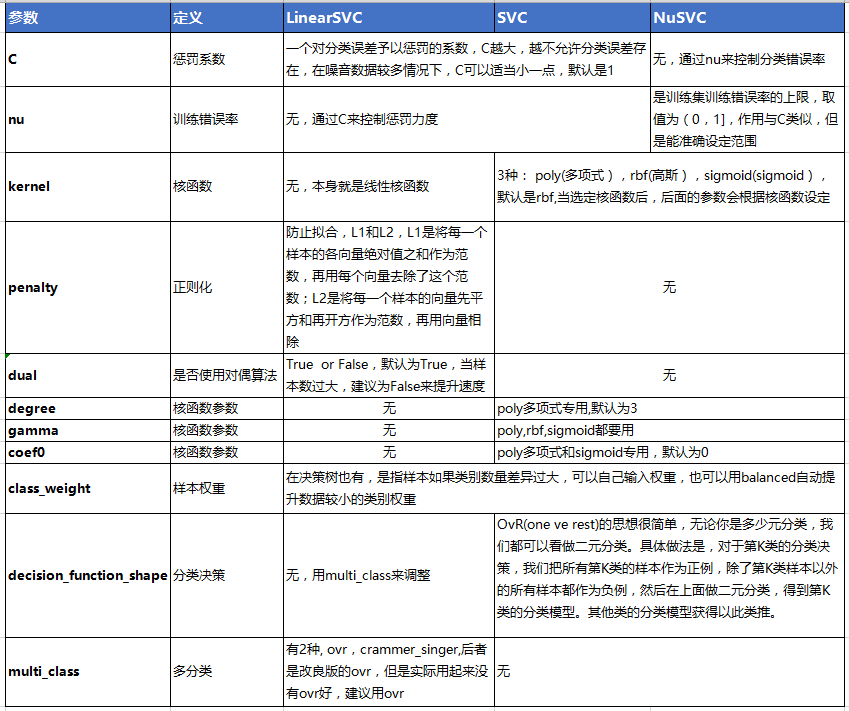二、回归

SVM回归算法库的重要参数大部分和分类算法库类似，因此这里重点讲述和分类算法库不同的部分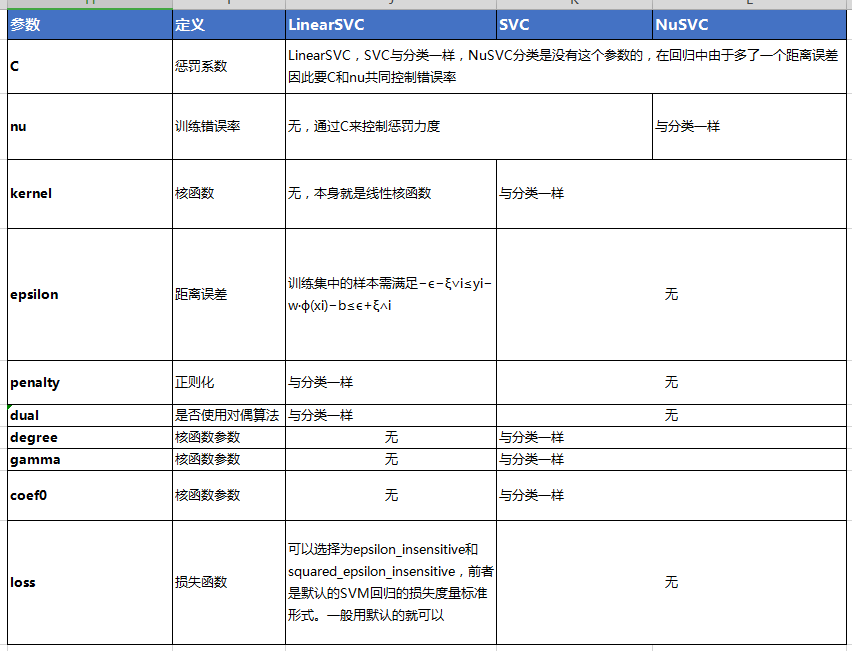三、参数注意事项

①对训练数据和测试数据最好都做归一化
②如果特征非常多，或者样本数远少于特征数时，数据更偏向线性可分，用线性核函数效果就会很好
③优先选择线性核函数，如果拟合不好同，再推荐默认的高斯（rbf),因为高斯需要通过交叉验证选择适合的惩罚系数C和gamma
④理论上高斯不会比线性核差，但是高斯需要花非常多的时间在调参上，实际业务中能用线性就用线性

四、GridSearchCV调参实例

① 用sklearn数据生成器生成特征数为2的圆环型数据

import numpy as np
import matplotlib.pyplot as plt
from sklearn import svm,datasets
from sklearn.svm import SVC
from sklearn.datasets import make_moons,make_circles,make_classification

X, y = make_circles(noise=0.2, factor=0.5, random_state=1) # make_circles是环形，noise是加一点噪音，factor是两个圆形的距离
from sklearn.preprocessing import StandardScaler
X = StandardScaler().fit_transform(X)  # StandardScaler 可以通过对训练集生成一个转换器，然后可以同时转化训练集和测试集，测试集直接用transform就好了

②使用线性核函数，默认参数

# 先使用线性核函数
clf = LinearSVC()
clf.fit(X,y)
print('线性核函数模型评分:',clf.score(X,y))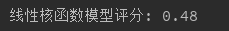在未调参情况下，评分非常低，只有0.48，调参如下：

from sklearn.model_selection import GridSearchCV
grid = GridSearchCV(LinearSVC(), param_grid={'C':np.linspace(0.1,10,50)}, cv=4) # SVC默认是高斯核，高斯核函数有2个参数c和gamma,CV 是将训练集分成多少从来进行交叉验证
grid.fit(X,y)
print('grid_best_params:',  grid.best_params_)
print('grid.best_score_:', grid.best_score_)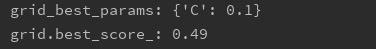调参后最佳C值为0.1，但是分数只提升了0.1，说明数据是线性不可分的，需要尝试其它非线性核函数，先分别对高斯，多项式和sigmoid核函数进行调参，结果如下：

param_grid=[{"kernel":["rbf"],"C":[0.1, 1, 10], "gamma": [1, 0.1, 0.01]},
{"kernel":["poly"],"C": [0.1, 1, 10], "gamma": [1, 0.1, 0.01],"degree":[3,5,10],"coef0":[0,0.1,1]},
{"kernel":["sigmoid"], "C": [0.1, 1, 10], "gamma": [1, 0.1, 0.01],"coef0":[0,0.1,1]}]

grid = GridSearchCV(SVC(), param_grid=param_grid, cv=4)
grid.fit(X,y)
print('grid_best_params:',  grid.best_params_)
print('grid.best_score_:', grid.best_score_)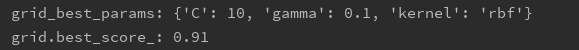最佳的核函数是高斯（rbf），且分数达到了0.91，相对线性核函数翻了一倍

为了更清楚地看到调参过程对分类的影响，对调参的分类结果进行可视化，先看下原始数据：

ax = plt.subplot()
ax.set_title('data')
ax.scatter(X[:, 0], X[:, 1], c=y, cmap=plt.cm.summer)
ax.set_xticks(())
ax.set_yticks(())
plt.tight_layout() #自动调节子图参数，使之填充整个区域
plt.show()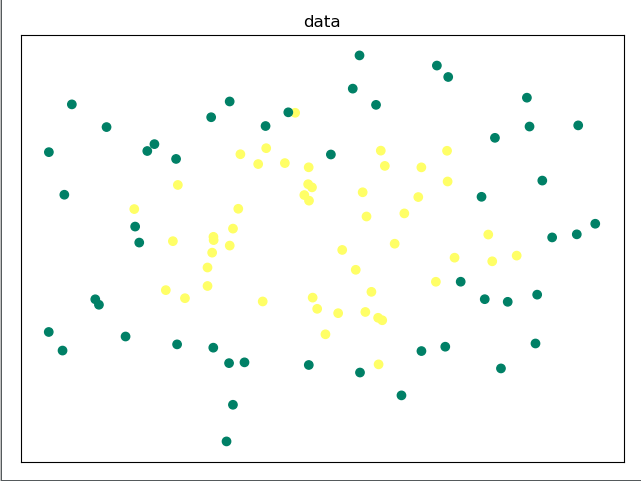for i, kernel in enumerate(('rbf','poly','sigmoid')):
for j ,C in enumerate((0.1, 1)):  # 对于一个序列或者列表，既要遍历索引又要遍历元素时,将下标和列表中的内容组成一个tutle().还可以enumerate(序列，1)，这样下标会从1开始
for k, gamma in enumerate((1, 0.1)):
plt.subplot()
clf = SVC(kernel=kernel, C=C, gamma=gamma)
clf.fit(X, y)
Z = clf.predict(np.c_[xx.ravel(), yy.ravel()]) # x.shape(3),y.shape(2),xx,yy.shape(2,3),np.c_,shape(6),xx = [[1,2,3],[3,5,7]],yy=[[2,3,4],[5,3,2]],xx.ravel()=[1,2,3,3,5,7],yy.ravel=[2,3,4,5,3,2],np.c_=[[1,2],[2,],[]]
Z = Z.reshape(xx.shape)
plt.contourf(xx, yy, Z, cmap=plt.cm.coolwarm, alpha=0.8)
plt.scatter(X[:, 0], X[:, 1], c=y, cmap=plt.cm.coolwarm)
plt.xlim(xx.min(), xx.max())
plt.ylim(yy.min(), yy.max())
plt.xlabel('kernel='+str(kernel)+' gamma=' + str(gamma) + ' C=' + str(C))
plt.show()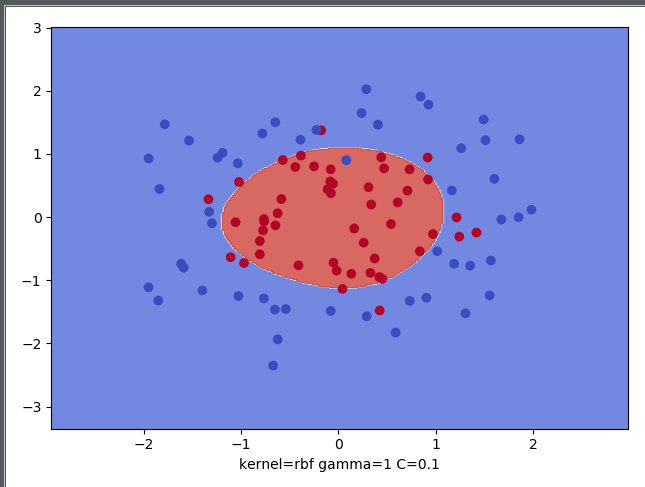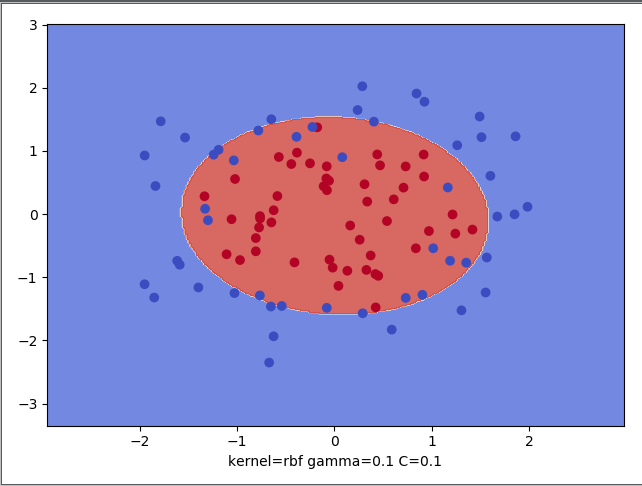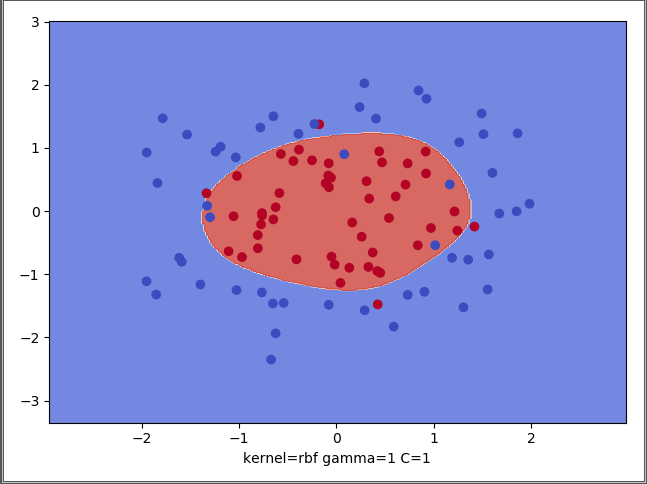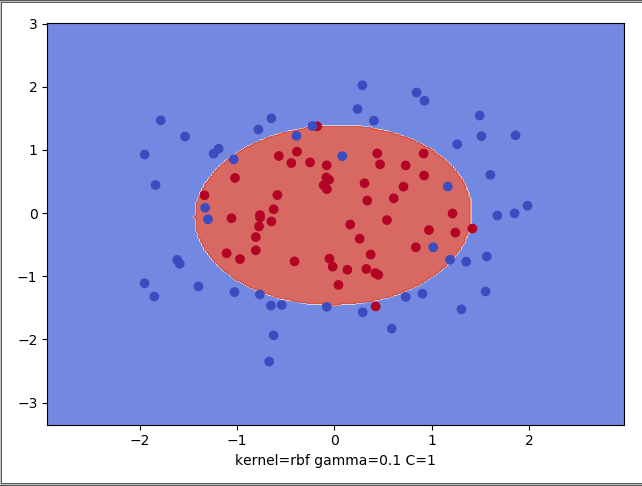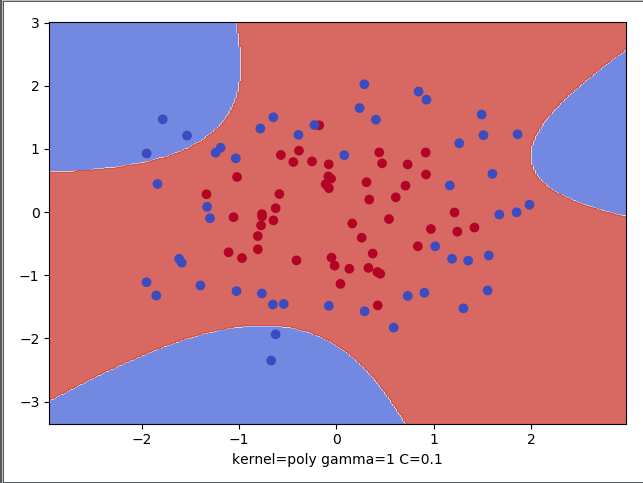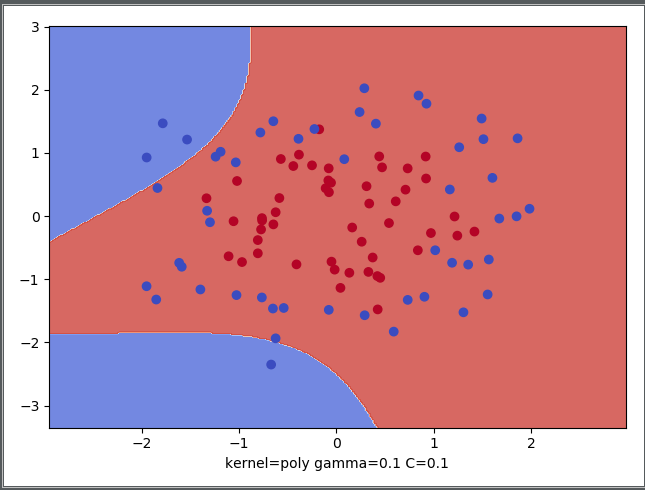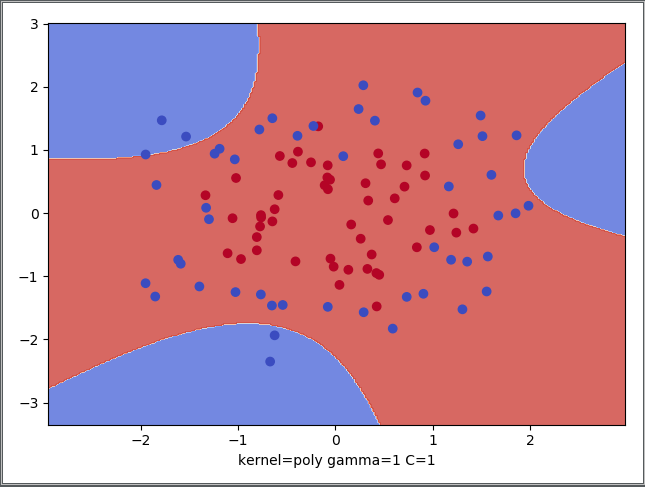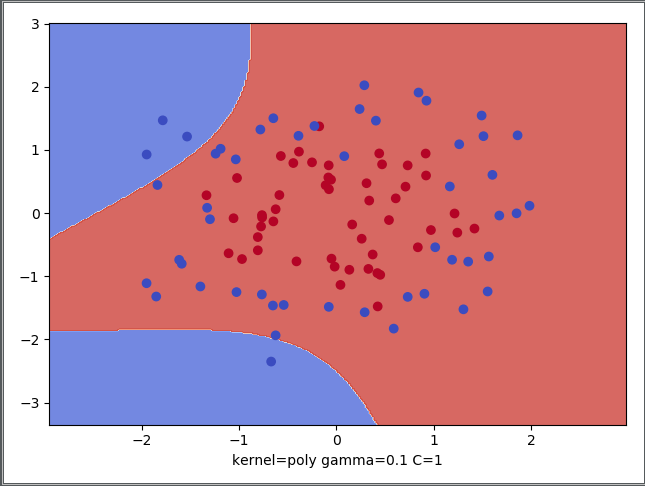由于原始数据是环形，在增加了噪音后基本偏向于圆形分布，因此高斯是更适合的。从图像上也能看出，高斯分类效果高于任一一张多项式分布的，这里加上一张sigmoid的，也是要比高斯差一些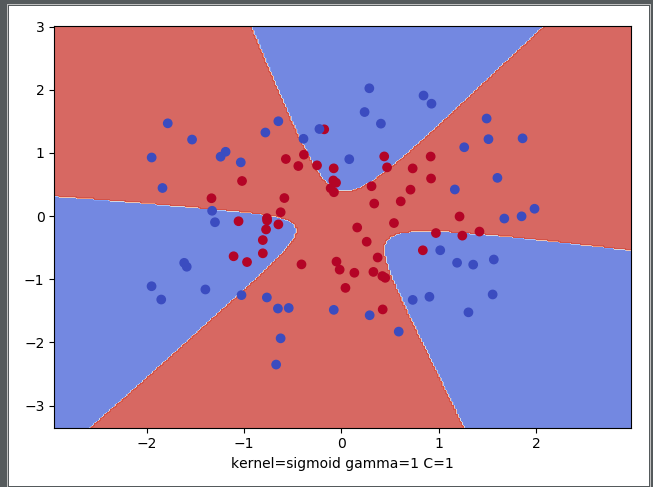本人互联网数据分析师，目前已出ExcelSQLPandasMatplotlibSeaborn机器学习统计学个性推荐关联算法工作总结系列。

微信搜索 " 数据小斑马" 公众号，回复“数据分析"就可以免费领取数据分析升级打怪 15本必备教材展开全文支持向量机 SVM调参 SVM核函数 SVM核函数调参
• 除了"linear"以外的核函数都能够处理非线性情况，那究竟什么时候选择哪一个核函数呢？遗憾的是，关于核函数在不同数据集上的研究甚少，谷歌学术上的论文中也没有几篇是研究核函数在SVM中的运用的，更多的是关于...机器学习 算法 SVM 核函数 python
• 线性支持向量机2.1 线性分类SVM的软间隔最大化2.2 软间隔最大化目标函数的优化2.3 线性分类SVM算法总结2.4 软间隔最大化时的支持向量 一.线性可分支持向量机存在的问题 有些时候样本中混入了异常点，导致不能线性...
• 线性分类问题 遇到分类问题的时候，最理想的状态，当然是样本在向量空间中都是线性可分的，我们可以清晰无误地把它们分隔成不同的类别——线性可分 SVM。 如果实在不行，我们可以容忍少数不能被正确划分，只要...
• 核函数详解

万次阅读 多人点赞 2018-04-17 21:11:28
这篇博文开始的例子就很好地揭示了核函数的简单原理，写得很好！原地址：https://blog.csdn.net/zkq_1986/article/details/524482381 核函数K（kernel function）定义核函数K（kernel function）就是指K(x, y) = &...
• 你知道什么是核函数吗

千次阅读 多人点赞 2020-03-03 11:04:52
1.线性核函数线性核函数（Linear Kernel）是多项式核函数的特例，优点是简洁，缺点是对线性不可分数据集没有解决办法。主要用于线性可分的情况，我们可以看到特征空间到输入空间的维度是一样的，其参数少速度快...机器学习 人工智能 深度学习 核函数 SVM
• RBF核函数和Linera核函数关系

千次阅读 2019-04-14 20:56:12
1.Linear 主要用于线性可分的情形。参数少，速度快，对于一般数据，分类效果已经很理想了。 2. RBF 主要用于线性不可分的情形。参数多，分类结果非常依赖于参数。通过训练数据的交叉验证来寻找合适的参数，验证...核函数 机器学习 人工智能
• 技术 回顾 上次讲到了SVM的对偶形式，这个对偶问题也是二次规划问题。所以可以使用二次规划的方法来解决。之所以要使用SVM的对偶形式，是因为它告诉了我们SVMSVM背后的一些几何意义，例如，对偶的SVM问题的解...SVM 机器学习
• 非线性SVM与核函数

千次阅读 2017-08-25 10:53:41
本文首先介绍核函数以及核技巧在SVM中的应用。然后介绍正定核概念和常用核函数。最后总结非线性SVM算法。SVM
• 或者说你在求解线性问题，而权重w恰好是训练样本的加权组合的情况下，都可以用上核函数的方法，来做到提升维度，进而完成非线性线性转换的一个目的。 可能有同学不理解，为什么从低维度升到高纬度就能够做到从...核函数
• SVM中的核函数

千次阅读 2017-04-18 17:04:00
1 核函数本质 ​核函数的本质可以概括为如下三点： 1）实际应用中，常常遇到线性不可分的情况​。针对这种情况，常用做法是把样例特征映射到高维空间中，转化为线性可分问题。 2）将样例特征映射到高维空间，可能...
• 首先，线性规划，比较简单，对误差方程 Ex = （y-mx-c）^2 分别求偏导，再令偏导等于0 一步一步的求出m和c。 令偏导等于0的原因是为了寻找极致点，我们假设每次迭代的都是极值点，而极值点的特点是导数为0。 ...机器学习

万次阅读 多人点赞 2016-05-26 21:47:44
1.核函数 1.1核函数的由来 -----------还记得为何要选用核函数么？----------- 对于这个问题，在Jasper's Java Jacal博客《SVM入门（七）为何需要核函数》中做了很详细的阐述，另外博主对于SVM德入门学习也是做了很...svm RBF 核函数
• 例题 判断 (−1+xTx′)(−1+xTx′)(-1 + x^Tx') 是不是核函数？ 3.3 常用的核函数 3.3.1 二次多项式核 3.3.2 高斯核 3.1 核技巧解决非线性SVM 3.1.1 非线性SVM解决思路 ...SVM
• SVM核函数概述

万次阅读 多人点赞 2019-03-27 08:55:53
咱们首先给出核函数的来头：在上文中，我们已经了解到了SVM处理线性可分的情况，而对于非线性的情况，SVM 的处理方法是选择一个核函数 κ(⋅,⋅) ，通过将数据映射到高维空间，来解决在原始空间中线性不可分的问题...SVM 支持向量机 核函数 机器学习 算法
• · 支持向量机：模型篇3–对偶问题的求解: SMO算法 · 支持向量机：模型篇4–核函数与非线性优化 · 支持向量机：模型篇5–向量机的软间隔拓展 ##代码篇 · 支持向量机：代码篇1-基于CVXPT优化函数求解 · 支持向量...支持向量机 核函数 拉格朗日对偶 非线性
•SVM 核函数
• 机器学习笔记之核函数

千次阅读 多人点赞 2018-06-18 15:43:45
线性核函数 多项式核函数 高斯核函数(RBF) sigmoid核函数 核函数的选取原则 基本概念 核函数定义： 核函数：是映射关系的内积，映射函数本身仅仅是一种映射关系，并没有增加维度的特性，不过可以利用核...核函数 机器学习
• SVM常用核函数

万次阅读 2018-07-18 15:44:39
核函数的作用是，通过将空间内线性不可分的数据映射到一个高维的特征空间，使得数据在特征空间内是可分的（动画）。我们定义这种映射为 ϕ(x)，那么我们就可以把求解约束最优化问题变为 minα−−&amp;gt...SVM Kernel 核函数 opencv 分类器
• 源码核函数

2016-01-10 13:41:39
源码核函数 Multi-Transfer:Transfer Learning with Multiple Views and Multiple Sources
• 为什么要有个核函数在对偶支持向量机中我们谈到要避开特征转换后高VC维度空间给我们带来的计算复杂度的影响。但是单单的对偶问题没有实现这一点，对偶问题只是让计算看起来避开了VC维度带来的影响，但是这个VC维度...核函数 高斯核函数 支持向量机的核函数 机器学习技法 林轩田...